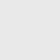# Quick Resistor Value Calculator: 4, 5, 6 Bands & Ohm’s Law

Whether you're a seasoned engineer or a beginner in electronics, comprehending how to read the resistor color code is a fundamental skill. This article delves into the significance of resistor color codes, their interpretation,

In the world of electronics, where precision is paramount, understanding the value of a resistor is essential. This is where the Resistor Color Code Calculator becomes your trusted companion.

Check out our amazing Resistor color code calculator. In this calculator, you will see the following:

• Band 4, 5, 6 Color code calculator
• Resistor Standard value E-series calculation
• Ohm’s Law voltage Calculation Tool

## Why Resistor Color Codes Matter

Resistors serve as the backbone of electronic circuits, regulating the flow of electric current. Identifying their values accurately is crucial for circuit design, troubleshooting, and repair. Rather than relying on printed values, which can fade or become illegible over time, the resistor color code offers a durable and universally recognized method for value identification.

~ If you want to know more about Resistors and Color code in-depth check out our Ultimate Guide to Resistor“.

## How to Read Resistor Color Code

Understanding the resistor color code is a skill that every electronics enthusiast should possess. This system encodes the resistance value, tolerance, and sometimes temperature coefficient of a resistor in a series of colored bands. Let’s break down how to decipher these bands:

### > Color Bands

Most resistors have either four, five, or six-colored bands. Each color corresponds to a digit or multiplier, and the bands are read from left to right.

### > First and Second Bands

These represent the significant digits of the resistance value. For example, if the first band is red and the second is green, it signifies a value of 25.

### > Multiplier Band

The third band indicates the multiplier, which multiplies the significant digits. A brown band in this position represents a multiplier of 10, giving us 250 ohms.

### > Tolerance Band (Optional)

The fourth band, if present, denotes the tolerance or allowable variation in the resistor’s value. A gold band implies a tolerance of ±5%, while a silver band indicates ±10%.

### > Temperature Coefficient Band (Optional)

In six-band resistors, the fifth band represents the temperature coefficient, indicating how much the resistor’s value changes with temperature.

## How to Use It?

Now that you understand the basics of resistor color codes, let’s explore how to utilize the Resistor Color Code Calculator effectively:

### 1. Select the Band Count

Start by choosing the number of bands on your resistor. Common options include four, five, and six bands.

### 2. Input the Colors

Enter the colors of each band, starting from the left. The calculator will then calculate the resistance value and provide you with the result.

### 3. Interpret the Result

The calculator will display the resistance value, tolerance (if applicable), and sometimes the temperature coefficient. This information allows you to quickly and accurately identify the resistor’s characteristics.

## In Summary

In conclusion, the Resistor Color Code Calculator is a valuable tool for anyone working with electronics. It simplifies the process of identifying resistor values and ensures the accuracy required for successful circuit design and repair. Understanding resistor color codes

## Subscribe to our weekly newsletter

Subscribe
Notify ofInline Feedbacks

## Browse## Browse